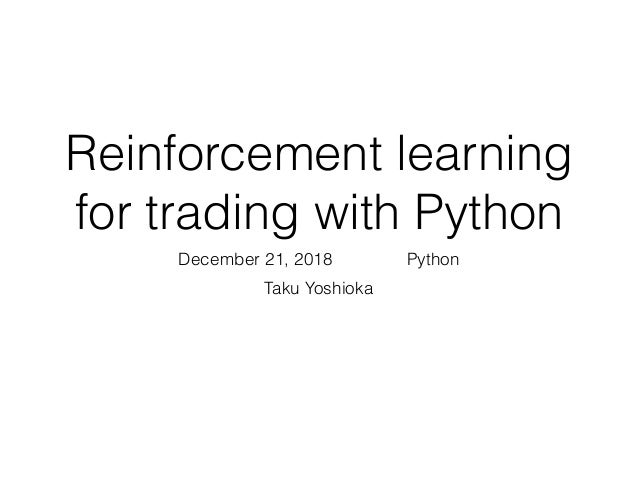Successfully reported this slideshow.Upcoming SlideShare
×

RL for learning trading strategy on a simple trading model. Presented at "京都はんなりPythonの会".

• Full Name
Comment goes here.

Are you sure you want to Yes No• Be the first to like this

1. 1. Reinforcement learning for trading with Python December 21, 2018 はんなりPython Taku Yoshioka
2. 2. • A previous work on github: q-trader • Q-learning • Trading model • State, action and reward • Implementation • Results
4. 4. edwardhdlu/q-trader • An implementation of Q-learning applied to (short- term) stock trading
5. 5. Action value function (Q-value) • Expected discounted cumlative future reward given the current state and action then following the optimal policy (mapping from state to action) Q(st, at) = E " X k=0 k rt+k | s = st, a = at # : discount factor t: time step st: state at: action rt: (immediate) reward
6. 6. Q-learning • An algorithm of Reinforcement learning for learning the optimal Q-value Qnew (st, at) = (1 ↵)Qold (st, at) + ↵(rt + max a Q(st+1, a)) ↵ 2 (0, 1): learning rate • For applying Q-learning, collect samples in episodes represented as tuples (st, at, rt, st+1)
7. 7. Deep Q-learning • Representing action value function with a deep network and minimizing loss function L = X t2D (Q(st, at) yt) 2 yt = rt + max a Q(st+1, a) Note • In contrast to supervised learning, the target value involves the current network outputs. Thus network parameters should be gradually updated • The order of samples in minibatches can be randomized to decorrelate sequential samples (replay buffer) D: minibatch
8. 8. Trading model • Based on closing prices • Three actions: buy (1 unit), sell (1 unit), sit • Ignoring transaction fee • Immediate transaction • Limited number of units we can keep
9. 9. State • State: n-step time window of the 1-step differences of past stock prices • Sigmoid function for input scaling issue st = (dt ⌧+1, · · · , dt 1, dt) dt = sigmoid (pt pt 1) pt: price at time t (discrete) ⌧: time window size (steps)
10. 10. Reward • Depending on action • Sit: 0 • Buy: a negative constant (conﬁgurable) • Sell: proﬁt (sell price - bought price) This needs to be appropriately designed
11. 11. Implementation • Training (train.py) Initialization, sample collection, training loop • Environment (environment.py) Loading stock data, making state transition with reward • Agent (agent.py) Feature extraction, Q-learning, e-greedy exploration, saving model • Results examination (learning_curve.py, plot_learning_curve.py, evaluate.py) Compute learning curve for given data, plotting
12. 12. Conﬁguration • For comparison of various experimental settings stock_name : ^GSPC window_size : 10 episode_count: 500 result_dir : ./models/config batch_size: 32 clip_reward : True reward_for_buy: -10 gamma : 0.995 learning_rate : 0.001 optimizer : Adam inventory_max : 10
13. 13. from ruamel.yaml import YAML with open(sys.argv) as f: yaml = YAML() config = yaml.load(f) stock_name = config["stock_name"] window_size = config["window_size"] episode_count = config["episode_count"] result_dir = config["result_dir"] batch_size = config["batch_size"] Parsing conﬁg ﬁle • Get parameters as a dict
14. 14. stock_name, window_size, episode_count = sys.argv, int(sys.argv), int( sys.argv) batch_size = 32 agent = Agent(window_size) # Environment env = SimpleTradeEnv(stock_name, window_size, agent) train.py • Instantiate RL agent and environment for trading
15. 15. # Loop over episodes for e in range(episode_count + 1): # Initialization before starting an episode state = env.reset() agent.inventory = [] done = False # Loop in an episode while not done: action = agent.act(state) next_state, reward, done, _ = env.step(action) agent.memory.append((state, action, reward, next_state, done)) state = next_state if len(agent.memory) > batch_size: agent.expReplay(batch_size) if e % 10 == 0: agent.model.save("models/model_ep" + str(e)) • Loop over episodes • Collect samples and train the model (expReplay) • Train model every 10 episodes